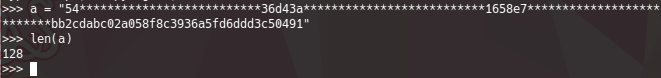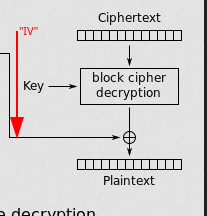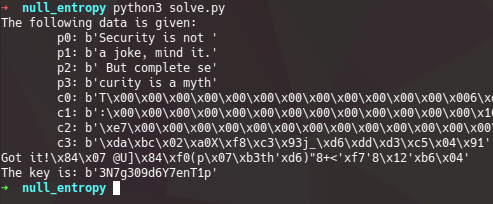# cyberhack{null entropy}

Hi there! The last week, we played on a CTF organized by `cyberhack`, was an amazing time, and got a happy 1st place as a team ❤️ !# Summary

The challenge name is “Null Entropy”, was a crypto - “hard” challenge, and a very fun to script :D

``````The management staff has constantly insisted Mr. Chris Brown Chandelier to induce maximum randomness into their security algorithms. He always denied it due to the budget problems, but we think he lacks knowledge of standard applications of symmetric ciphers. Can you show him what problem his actions can produce?

https://github.com/bittentech/cyberhackctf/tree/master/Null%20Entropy

NOTE: The '*' character in the string means the correct byte is redacted.

Author: Ansh Bhawnani

400

``````

We got 2 files: the `encrypt.py` script, and the `ciphertext.txt`

## encrypt.py

``````from Crypto.Cipher import AES
import binascii, sys
from flag import flag

key = "3N7g309d6Y7enT**"
IV = flag

message = 'Security is not a joke, mind it. But complete security is a myth'

def encrypt(message,passphrase):
aes = AES.new(passphrase, AES.MODE_CBC, IV)
return aes.encrypt(message)

print("Encrypted data: ", binascii.hexlify(encrypt(message,key)).decode())
``````

## ciphertext.txt

``````TlRRcUtpb3FLaW9xS2lvcUtpb3FLaW9xS2lvcUtpb3FLaW9xS2pNMlpEUXpZU29xS2lvcUtpb3FLaW9xS2lvcUtpb3FLaW9xS2lvcUtpb3FNVFkxT0dVM0tpb3FLaW9xS2lvcUtpb3FLaW9xS2lvcUtpb3FLaW9xS2lwaVlqSmpaR0ZpWXpBeVlUQTFPR1k0WXpNNU16WmhOV1prTm1Sa1pETmpOVEEwT1RFPQ==
-- double base64 decode --
54**************************36d43a**************************1658e7**************************bb2cdabc02a058f8c3936a5fd6ddd3c50491
``````

From here, we can see that the `ciphertext.txt` is 64 bytes long (128 hex values), which means, that we will going to deal with 16 bytes blocks, and 4 blocks in total (since the `plaintext` is 64 bytes long).From the `encrypt.py` script, we can see that the flag is the `IV` asigned to the `AES CBC 128 encryption`. We got the `AES CBC key`, but we dont know the last 2 bytes from it.

So, we know that the `AES CBC` encryption goes like this:If we take the `encryption box` as a `black box`, we know that to get the `IV` (flag), we have to know the first block of the `ciphertext`, but the only thing that we know, is the `entire last block of ciphertext`, and a couple of bytes of the others.
With this information, the first thing that we need to do, is know the correct key, we just need to bruteforce 2 bytes.
Then, we have to start to find the `correct values` of the previous ciphertexts blocks (We must do the reverse operation to the encryption, the decryption, LOL). If we pay attention to the diagram, specific, the `last decryption block`.We can let the `ongoing previous ciphertext block` be the “IV vector”, an “IV” that is just the “previous ciphertext block”, so, with this in mind, we can take Cn-1 ciphertext, `XOR` it with the `decrypted block`, since we have the `plain text`, start to bruteforce, byte by byte the `"IV"` (which is just the previous cipher block), and start to assemble the entire `known plaintext`.
So, the attack vector is:

• Brute force the key (2 bytes)
• Start the decryption block by block in reverse order, to start to get the complete ciphertext.
• Once the entire `ciphertext` is known, brute the `IV` which is the flag.
• Get the flag (points 👀)

# solve.py

From here, we can craft a `skeleton` for the solution.

``````from Crypto.Cipher import AES
import binascii, sys
import time
#from flag import flag

key = b"3N7g309d6Y7enT**"
#IV = flag
IV = b"cyberhack{test_}"

ciphertext = b"54**************************36d43a**************************1658e7**************************bb2cdabc02a058f8c3936a5fd6ddd3c50491"

message = b'Security is not a joke, mind it. But complete security is a myth'

'''
The following data is given:
p0: b'Security is not '
p1: b'a joke, mind it.'
p2: b' But complete se'
p3: b'curity is a myth'
c0: 54**************************36d4
c1: b'3a**************************1658'
c2: b'e7**************************bb2c'
c3: b'\xda\xbc\x02\xa0X\xf8\xc3\x93j_\xd6\xdd\xd3\xc5\x04\x91'

'''

def encrypt(message,passphrase):
aes = AES.new(passphrase, AES.MODE_CBC, IV)
return aes.encrypt(message)

# We must to brute 2 bytes from the key
key = b"3N7g309d6Y7enT"

# Each block of each message
p0 = message[:16]
p1 = message[16:32]
p2 = message[32:48]
p3 = message[48:64]

# Each block of ciphertext
c0 = b'\x54\x00\x00\x00\x00\x00\x00\x00\x00\x00\x00\x00\x00\x00\x36\xd4'
c1 = b'\x3a\x00\x00\x00\x00\x00\x00\x00\x00\x00\x00\x00\x00\x00\x16\x58'
c2 = b'\xe7\x00\x00\x00\x00\x00\x00\x00\x00\x00\x00\x00\x00\x00\xbb\x2c'
c3 = b'\xda\xbc\x02\xa0X\xf8\xc3\x93j_\xd6\xdd\xd3\xc5\x04\x91'

print("""The following data is given:
p0: {}
p1: {}
p2: {}
p3: {}
c0: {}
c1: {}
c2: {}
c3: {}""".format(p0, p1, p2, p3, c0, c1, c2, c3))

``````

The only thing that I did here, was to cut the `encrypt.py`, and add all the info that we have for the moment (I replaced the `*`, for `\x00` to pad).

So, according to the first objective, is to bruteforce the key. We can do this, by bruteforcing byte by byte (just 2 bytes), since we have the entire last `ciphertext block`, and the `first and last 2 bytes` of the `previous ciphertext block`, we can bruteforce those values.

``````from Crypto.Cipher import AES
import binascii
import sys
import time
#from flag import flag

key = b"3N7g309d6Y7enT**"
#IV = flag
IV = b"cyberhack{test_}"

ciphertext = b"54**************************36d43a**************************1658e7**************************bb2cdabc02a058f8c3936a5fd6ddd3c50491"

message = b'Security is not a joke, mind it. But complete security is a myth'

'''
The following data is given:
p0: b'Security is not '
p1: b'a joke, mind it.'
p2: b' But complete se'
p3: b'curity is a myth'
c0: 54**************************36d4
c1: b'3a**************************1658'
c2: b'e7**************************bb2c'
c3: b'\xda\xbc\x02\xa0X\xf8\xc3\x93j_\xd6\xdd\xd3\xc5\x04\x91'

'''

def encrypt(message, passphrase):
aes = AES.new(passphrase, AES.MODE_CBC, IV)
return aes.encrypt(message)

key = b"3N7g309d6Y7enT"
p0 = message[:16]
p1 = message[16:32]
p2 = message[32:48]
p3 = message[48:64]

c0 = b'\x54\x00\x00\x00\x00\x00\x00\x00\x00\x00\x00\x00\x00\x00\x36\xd4'
c1 = b'\x3a\x00\x00\x00\x00\x00\x00\x00\x00\x00\x00\x00\x00\x00\x16\x58'
c2 = b'\xe7\x00\x00\x00\x00\x00\x00\x00\x00\x00\x00\x00\x00\x00\xbb\x2c'
c3 = b'\xda\xbc\x02\xa0X\xf8\xc3\x93j_\xd6\xdd\xd3\xc5\x04\x91'

print("""The following data is given:
p0: {}
p1: {}
p2: {}
p3: {}
c0: {}
c1: {}
c2: {}
c3: {}""".format(p0, p1, p2, p3, c0, c1, c2, c3))

def get_key():
end = False
# We bruteforce the last 2 bytes
for k in range(0xff):
if end == True:
break
for j in range(0xff):
b1 = bytes([k])
b2 = bytes([j])
key_fmt = key + b1 + b2

# We use the `last - 1 ` block as a IV vector of decryption
# Since its just an xor, and we know the first and last 2 bytes of the 'last - 1' ciphertext, we can get those correct values.
aes = AES.new(key_fmt, AES.MODE_CBC, c2)
cx = aes.decrypt(c3)

c2_1 = c2[:1]
c2_2 = c2[-2:-1]
c2_3 = c2[-1:]
print(cx, end='\r')
# If the
if cx.startswith(b'c') and cx.endswith(b'th'):
print("Got it!")
print("The key is: {}".format(key_fmt))
return key_fmt
#        if c2[2:] ==

if __name__ == "__main__":
key = get_key()

``````Nice! we got the correct key to make the decryption. Now, we have to brute byte by byte the entire `"IV"` value for each block, which in the blocks that are not the first one, is just the previous `cipher block`

I made up this function to brute every ciphertext block, I’ll try to make the code self explanatory, ping me if there’s any doubt.

``````
from Crypto.Cipher import AES
import binascii
import sys
import time
#from flag import flag

key = b"3N7g309d6Y7enT**"
#IV = flag
IV = b"cyberhack{test_}"

ciphertext = b"54**************************36d43a**************************1658e7**************************bb2cdabc02a058f8c3936a5fd6ddd3c50491"

message = b'Security is not a joke, mind it. But complete security is a myth'

'''
The following data is given:
p0: b'Security is not '
p1: b'a joke, mind it.'
p2: b' But complete se'
p3: b'curity is a myth'
c0: 54**************************36d4
c1: b'3a**************************1658'
c2: b'e7**************************bb2c'
c3: b'\xda\xbc\x02\xa0X\xf8\xc3\x93j_\xd6\xdd\xd3\xc5\x04\x91'

'''

def encrypt(message, passphrase):
aes = AES.new(passphrase, AES.MODE_CBC, IV)
return aes.encrypt(message)

key = b"3N7g309d6Y7enT"
p0 = message[:16]
p1 = message[16:32]
p2 = message[32:48]
p3 = message[48:64]

c0 = b'\x54\x00\x00\x00\x00\x00\x00\x00\x00\x00\x00\x00\x00\x00\x36\xd4'
c1 = b'\x3a\x00\x00\x00\x00\x00\x00\x00\x00\x00\x00\x00\x00\x00\x16\x58'
c2 = b'\xe7\x00\x00\x00\x00\x00\x00\x00\x00\x00\x00\x00\x00\x00\xbb\x2c'
c3 = b'\xda\xbc\x02\xa0X\xf8\xc3\x93j_\xd6\xdd\xd3\xc5\x04\x91'

print("""The following data is given:
p0: {}
p1: {}
p2: {}
p3: {}
c0: {}
c1: {}
c2: {}
c3: {}""".format(p0, p1, p2, p3, c0, c1, c2, c3))

def get_key():
end = False
# We bruteforce the last 2 bytes
for k in range(0xff):
if end == True:
break
for j in range(0xff):
b1 = bytes([k])
b2 = bytes([j])
key_fmt = key + b1 + b2

# We use the `last - 1 ` block as a IV vector of decryption
# Since its just an xor, and we know the first and last 2 bytes of the 'last - 1' ciphertext, we can get those correct values.
aes = AES.new(key_fmt, AES.MODE_CBC, c2)
cx = aes.decrypt(c3)

c2_1 = c2[:1]
c2_2 = c2[-2:-1]
c2_3 = c2[-1:]
print(cx, end='\r')
# If the decrypt start and end with the known value, we found the key
if cx.startswith(b'c') and cx.endswith(b'th'):
print("Got it!")
print("The key is: {}".format(key_fmt))
return key_fmt

def decrypt_cipherblock(c_IV, c2decrypt, plaintext, last=False):
#print("Starting to decrypting the ciphertext: {}".format(c_IV))
# bruted ciphertext
# We start with the first byte of the "desire brute ciphertext"
b_block = bytes([c_IV])
# Counter of where we are on the ciphertext
counter = 2

fin = False
while not fin:
for k in range(0xff):
# We append the bruted block that we have for the moment to the current IV
to_use = b_block
# If the len of used IV is 16 bytes, we are done
if(len(to_use)) == 16:
fin = True
break
# If not, we append the forloop bytes
to_use += bytes([k])
# We append the left bytes of the ciphertext block
# remember that we are bruting byte by byte, like:
# and goon
to_use += c_IV[counter:]
# We make the AES object with our IV
aes = AES.new(key, AES.MODE_CBC, to_use)
# We decrypt the cipher text that we want
dec = aes.decrypt(c2decrypt)
print("{}".format(dec), end ='\r')
# If we got the correct byte of the plaintext, we move on to the next byte
if dec.startswith(plaintext[0:counter]):
if not last:
print(dec +b" "*20, end="\r",flush=True)
else:
print(b_block  + b" "*20, end="\r", flush=True)

counter = counter + 1
b_block = b_block + bytes([k])
time.sleep(.1)
break
print("",end="\n")
return b_block

if __name__ == "__main__":
key = get_key()
# The entires cipherblocks
# First, we brute the cipher block 2
c_e2 = decrypt_cipherblock(c2, c3, p3)
#print(c_e2)
time.sleep(2)
# brute the cipher block 1
c_e1 = decrypt_cipherblock(c1,c_e2, p2 )
#print(c_e1)
time.sleep(2)
# brute the cipher block 0 (sorry for the number)
c_e0 = decrypt_cipherblock(c0, c_e1, p1)
#print(c_e0)
time.sleep(2)
print("Starting to brute the pigmy block and hopefully got the IV (flag)")
time.sleep(1)
# Now we have the entire ciphertext, we just need to brute the IV!
# So we fill the next 15 bytes with junk
iv = b'{' + b'A' * 15
iv = decrypt_cipherblock(iv, c_e0, p0, last=True)
print("Got the flag")
print("cyberhack{}".format(iv.decode()))

``````

and yay, the flag `cyberhack{W3ak_IV_5uckS5}`

So hackish see the brute byte by byte 😂

hopefully this make sense to solve the challenge, or something related to this…

Cheers!

• f4d3